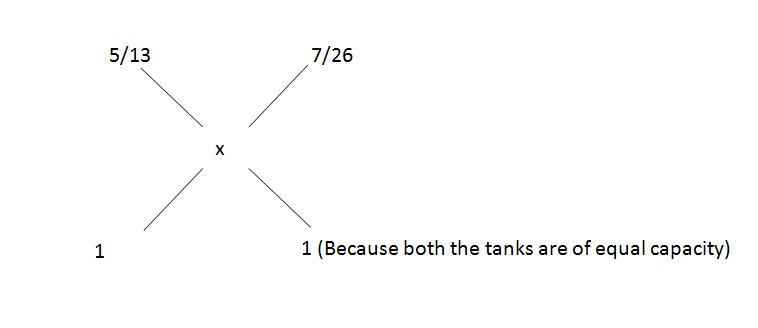# CAT Quantitative Aptitude Questions | CAT Ratios, Mixtures, Alligations and Averages Questions

###### CAT Questions | Mixtures and Alligations | Alligation

The question is from CAT Ratio, Mixtures and Averages. It is a simple question. When two mixures are mixed, what would be the ratio in the resultant mixture? CAT exam is known to test on basics rather than high funda ideas. A range of CAT questions can be asked from Ratios and Proportions, Mixtures, Alligations and Averages. Make sure you master the topics. 2IIMs CAT questions bank provides you with CAT questions that can help you gear for CAT Exam CAT 2020.

Question 34: Two tanks of similar volume are full of a mixture of oil and water. In the first, the ratio of oil and water is 5:8 and in the second, it is 7:19. If both these tanks are poured in a larger tank, what would be the resultant ratio of oil and water?

1. 1:3
2. 17:52
3. 151:304
4. 17:35

## Best CAT Coaching in Chennai

### Explanatory Answer

##### Method of solving this CAT Question from Ratio, Mixtures and Averages: When we mix two mixtures in a particular ratio, we get a third mixture. Given the third mixture how does one find the ratio in which they were mixed.

In this type of questions, take one of the components and find its proportion in the overall mixture.
In first tank, Oil/Water = $$frac{5}{8}\\$ therefore, Oil/Mixture = $\frac{5}{13}\\$ In second tank, Oil/Water = $\frac{7}{19}\\$ therefore, Oil/Mixture = $\frac{7}{26}\\$ Therefore,=> $\frac{7}{26}\\$ – x = x – $\frac{5}{13}\\$ => $\frac{7}{26}\\$ + $\frac{5}{13}\\$ = 2x => 2x = $\frac{$7 + 10$}{26}$ => x = $\frac{17}{52}\\$ Therefore, Oil : Mixture = $\frac{17}{52}\\$ => Oil : Water = $\frac{17}{$52-17$}$ = $\frac{17}{35}\\$ The question is "If both these tanks are poured in a larger tank, what would be the resultant ratio of oil and water?" ##### Hence, the answer is "17:35". Choice D is the correct answer. ###### Best CAT Online Coaching Try upto 40 hours for free Learn from the best! ###### Already have an Account? ###### CAT Coaching in ChennaiCAT 2021Enroll at 49,000/- 44,000/- Online Classroom Batches Starting Now! ###### Best CAT Coaching in Chennai Introductory offer of 5000/- Attend a Demo Class ###### Best Indore IPM & Rohtak IPM CoachingSignup and sample 9 full classes for free. Register now! ##### Where is 2IIM located? 2IIM Online CAT Coaching A Fermat Education Initiative, 58/16, Indira Gandhi Street, Kaveri Rangan Nagar, Saligramam, Chennai 600 093 ##### How to reach 2IIM? Phone:$91) 44 4505 8484
Mobile: (91) 99626 48484
WhatsApp: WhatsApp Now
Email: prep@2iim.com